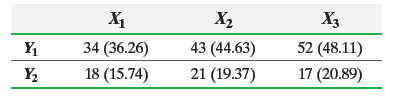×
Get Full Access to Statistics: Informed Decisions Using Data - 5 Edition - Chapter 12.2 - Problem 3
Get Full Access to Statistics: Informed Decisions Using Data - 5 Edition - Chapter 12.2 - Problem 3

×

# ?The following table contains observed values and expected values in parentheses for two categorical variables, X and Y, where variable X has three catISBN: 9780134133539 240

## Solution for problem 3 Chapter 12.2

Statistics: Informed Decisions Using Data | 5th Edition

• Textbook Solutions
• 2901 Step-by-step solutions solved by professors and subject experts
• Get 24/7 help from StudySoup virtual teaching assistantsStatistics: Informed Decisions Using Data | 5th Edition

4 5 1 303 Reviews
25
0
Problem 3

The following table contains observed values and expected values in parentheses for two categorical variables, X and Y, where variable X has three categories and variable Y has two categories:(a) Compute the value of the chi-square test statistic.

(b) Test the hypothesis that X and Y are independent at the= 0.05 level of significance.

Step-by-Step Solution:

Step 1 of 5) The following table contains observed values and expected values in parentheses for two categorical variables, X and Y, where variable X has three categories and variable Y has two categories: (a) Compute the value of the chi-square test statistic. (b) Test the hypothesis that X and Y are independent at the = 0.05 level of significance. Approach II: Compute the probability that both circuits are not defective and use the Complement Rule to determine the probability of at least 1 defective. We will illustrate both approaches. Solution Of the 100 circuits, 5 are defective, so 95 are not defective. Approach I: Construct a tree diagram to determine the possible outcomes of the experiment. See Figure 12 on the following page, where D stands for defective and G stands for good (not defective). Because the outcomes are not equally likely, we include the probabilities in our diagram to show how the probability of each outcome is obtained. (Multiply the individual probabilities along the corresponding path in the diagram.)

Step 2 of 2

##### ISBN: 9780134133539

The answer to “?The following table contains observed values and expected values in parentheses for two categorical variables, X and Y, where variable X has three categories and variable Y has two categories: (a) Compute the value of the chi-square test statistic.(b) Test the hypothesis that X and Y are independent at the = 0.05 level of significance.” is broken down into a number of easy to follow steps, and 55 words. Since the solution to 3 from 12.2 chapter was answered, more than 217 students have viewed the full step-by-step answer. Statistics: Informed Decisions Using Data was written by and is associated to the ISBN: 9780134133539. This full solution covers the following key subjects: . This expansive textbook survival guide covers 88 chapters, and 2422 solutions. This textbook survival guide was created for the textbook: Statistics: Informed Decisions Using Data, edition: 5. The full step-by-step solution to problem: 3 from chapter: 12.2 was answered by , our top Statistics solution expert on 01/15/18, 03:19PM.

## Discover and learn what students are asking

Calculus: Early Transcendental Functions : Increasing and Decreasing Functions and the First DerivativeTest
?Using a Graph In Exercises 3-8, use the graph to estimate the open intervals on which the function is increasing or decreasing. Then find the open int

Calculus: Early Transcendental Functions : First-Order Linear Differential Equations
?In Exercises 5-14, solve the first-order linear differential equation. $$\frac{d y}{d x}+\left(\frac{2}{x}\right) y=3 x-5$$

Calculus: Early Transcendental Functions : Cylindrical and Spherical Coordinates
?In Exercises 1-6, convert the point from cylindrical coordinates to rectangular coordinates. (-7, 0, 5)

Unlock Textbook Solution